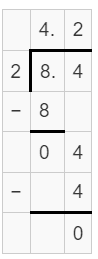# Decimal Division Worksheets

• ###### Decimal Division Word Problems

Decimal division worksheets are an essential educational tool designed to provide students with a structured and systematic means of learning and practicing the division of decimal numbers. These worksheets are comprised of numerous problems and exercises that progressively improve a student’s understanding of decimal division, thereby enhancing their overall mathematical proficiency.

When discussing decimal division worksheets, it’s important to acknowledge that they’re not a one-size-fits-all solution. They come in various types, tailored to different proficiency levels and learning objectives. The variety of exercises on these worksheets provides a comprehensive and in-depth approach to learning, ensuring that students grasp the fundamentals before moving onto more complex problems.

Basic Decimal Division – These worksheets contain simple exercises where students divide decimal numbers by whole numbers, e.g., 0.5 ÷ 5. These problems are designed to give students a basic understanding of how to divide decimals.

Division by Decimals – These exercises are a bit more advanced, as both the divisor and dividend are decimals. For example, a problem might be 0.56 ÷ 0.8. These exercises help students gain a deeper understanding of how to divide with decimals in both the dividend and divisor.

Word Problems – These exercises are given in a real-world context, which encourages students to apply their decimal division skills to solve problems. For instance, if a person has \$0.75 and wants to buy candy bars that cost \$0.15 each, how many candy bars can they purchase?

Decimal Division with Remainders – Some worksheets include problems where the division operation results in a remainder. For instance, when 1.4 is divided by 0.3, it leaves a remainder. These exercises are excellent for teaching students about the concept of remainders in decimal division.

Long Division with Decimals – These exercises require students to implement the long division method, a step-by-step procedure, with decimal numbers. These problems might initially appear complex but are crucial for students to understand the process of division in detail.

The comprehensive nature of these decimal division worksheets allows for a variety of benefits that improve a student’s overall math skills. First, they provide a structured framework for learning, allowing students to grasp the core principles of decimal division systematically. This structure helps improve the mathematical comprehension of students, enabling them to tackle more complex problems.

These worksheets will help you develop problem-solving skills. The ability to solve decimal division problems requires understanding, planning, and execution – skills that are not just limited to mathematics but are applicable to all areas of learning.

These exercises improve numerical fluency. With regular practice, students develop a sense of numbers and operations, aiding in quick calculations and estimations, a necessary skill for various math-related tasks.

The skills gained from decimal division worksheets have many real-world applications. From dividing monetary values, calculating rates, partitioning quantities, to understanding data in decimal forms in subjects like science or statistics, decimal division is a fundamental skill. For instance, if you’re shopping and need to divide the total cost between friends, or if you’re cooking and need to divide the quantities of ingredients, you would use decimal division.

### How Do You Divide Two Decimal Values?

Dividing two decimal values might seem intimidating at first, but the process is actually quite similar to ordinary long division. Here is a step-by-step process to guide you through it:

Step 1) Make the Divisor a Whole Number

If your divisor (the number you are dividing by) is a decimal, the first step is to make it a whole number. To do this, count the number of decimal places in the divisor and shift the decimal point to the right until it becomes a whole number. You then need to do the same with your dividend (the number you are dividing).

For example, let’s take the division problem 0.84 ÷ 0.2. The divisor is 0.2 and has one decimal place. You’d move the decimal point one place to the right, making the divisor 2. Now, you must do the same to the dividend, so 0.84 becomes 8.4. So, the division problem becomes 8.4 ÷ 2.Step 2) Perform Division as Usual

Now you perform the division as if you are dividing by a whole number. In our example, 8.4 ÷ 2 equals 4.2.